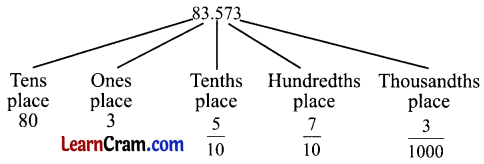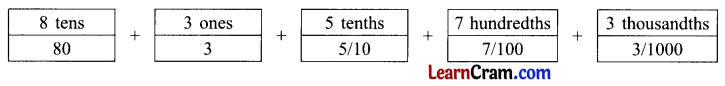# DAV Class 5 Maths Chapter 5 Worksheet 4 Solutions

The DAV Class 5 Maths Book Solutions Pdf and DAV Class 5 Maths Chapter 5 Worksheet 4 Solutions of Decimals offer comprehensive answers to textbook questions.

## DAV Class 5 Maths Ch 5 Worksheet 4 Solutions

Question 1.
Fill in the blanks. The first one is done for you.
(a) 17.25 : 2 is in the _________ place.
Solution:
tenths

(b) 892.416 : 4 is in the _________ place.
Solution:
tenths

(c) 57.63 : 3 is in the _________ place.
Solution:
hundredths

(d) 908.007 : 7 is in the _________ place.
Solution:
thousandths(e) 57.98 : 5 is in the _________ place.
Solution:
tens

(f) 139.081 : 0 is in the _________ place.
Solution:
tenths

Question 2.
Complete each of the following. The first one is done for you.
(a) 19.892 = _________
Solution:
19.892 = 10 + 9 + $$\frac{8}{10}+\frac{9}{100}+\frac{2}{1000}$$

(b) 15.217 = _________
Solution:
15.217 = 10 + 5 + $$\frac{2}{10}+\frac{1}{100}+\frac{7}{1000}$$

(c) 62.306 = _________
Solution:
62.306 = 60 + 2 + $$\frac{3}{10}+\frac{6}{1000}$$

(d) 149.356 = _________
Solution:
149.356 = 100 + 40 + 9 + $$\frac{3}{10}+\frac{5}{100}+\frac{6}{1000}$$(e) 762.027 = _________
Solution:
762.027 = 700 + 60 + 2 + $$\frac{2}{100}+\frac{7}{1000}$$

(f) 30.108 = _________
Solution:
30.108 = 30 + $$\frac{1}{10}+\frac{8}{1000}$$

Question 3.
Write in the expanded form. The first one is done for you.
(a) 92.73
Solution:
92.73 = 9 tens + 2 ones + 7 tenths + 3 hundredths
= 90 + 2 + $$\frac{7}{10}+\frac{3}{100}$$

(b) 14.657
Solution:
14.657 = 1 tens + 4 ones + 6 tenths + 5 hundredths + 7 thousandths
= 10 + 4 + $$\frac{6}{10}+\frac{5}{100}+\frac{7}{1000}$$

(c) 803.316
Solution:
803.316 = 8 hundreds + 0 tens + 3 ones + 3 tenths + 1 hundredths + 6 thousandths
= 800 + 3 + $$\frac{3}{10}+\frac{1}{100}+\frac{6}{1000}$$

(d) 24.405
Solution:
24.405 = 2 tens + 4 ones + 4 tenths + 0 hundredths + 5 thousandths
= 20 + 4 + $$\frac{4}{10}+\frac{5}{1000}$$(e) 127.253
Solution:
127.253 = 1 hundreds + 2 tens + 7 ones + 2 tenths + 5 hundredths + 3 thousandths
= 100 + 20 + 7 + $$\frac{2}{10}+\frac{5}{100}+\frac{3}{1000}$$

(f) 453.762
Solution:
453.762 = 4 hundreds + 5 tens + 3 ones + 7 tenths + 6 + hundredths + 2 thousandths
= 400 + 50 + 3 + $$\frac{7}{10}+\frac{6}{100}+\frac{2}{1000}$$

(g) 15.006
Solution:
15.006 = 1 tens + 5 ones + 0 tenths + 0 hundredths + 6 thousandths
= 10 + 5 + $$\frac{6}{1000}$$

Question 4.
Write the decimal form of each of the following.
(a) 5 + $$\frac{2}{10}+\frac{3}{100}+\frac{7}{1000}$$ = _________
Solution:
5.237

(b) 70 + 3 + $$\frac{9}{10}+\frac{8}{1000}$$ = _________
Solution:
6.045

(c) 6 + $$\frac{4}{100}+\frac{5}{1000}$$ = _________
Solution:
73.908(d) 200 + 5 + $$\frac{3}{100}+\frac{6}{1000}$$ = _________
Solution:
205.036

DAV Class 5 Maths Chapter 5 Worksheet 4 Notes

Expanded Form of a DecimalThe number can be written as:This is an expanded form of 83.573